# Intro to deep learning¶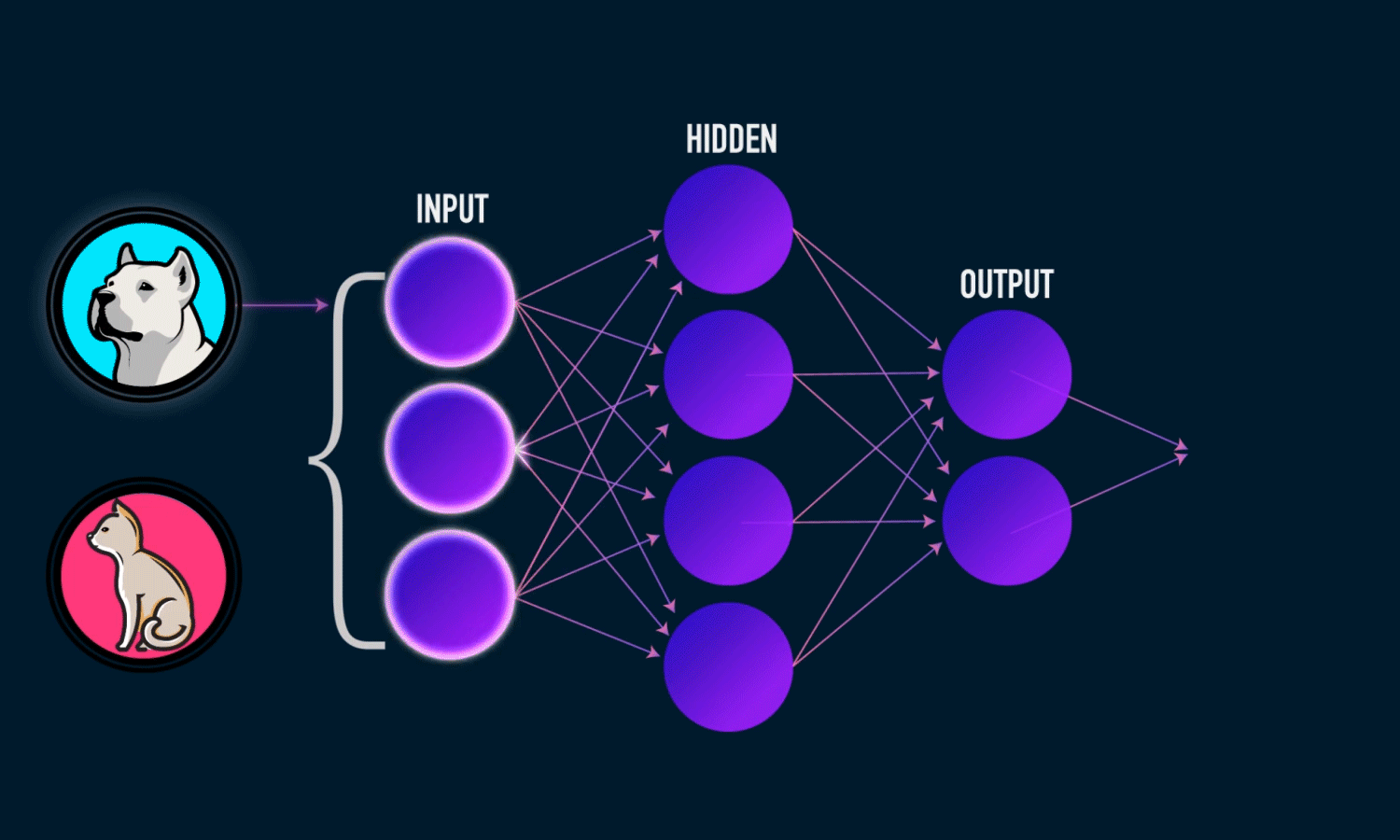Deep learning is an artificial intelligence function that closely resembles the workings of the human brain, processing data and finding patterns for use in decision making. Deep learning is a subset of machine learning in artificial intelligence (AI) that has networks capable of learning unsupervised from data that is unstructured or unlabeled. Also known as deep neural learning or deep neural network.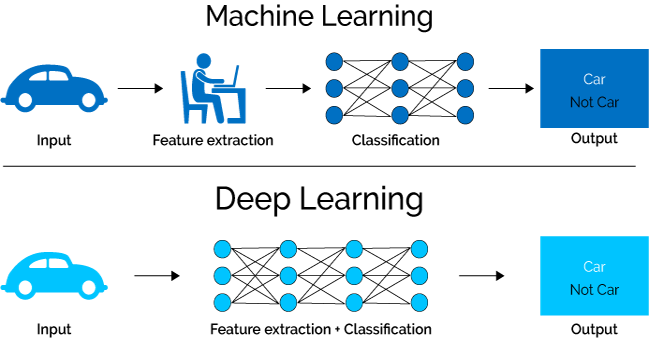One of the key differences between machine learning and deep learning is the feature extraction. Feature extraction is done by a human in machine learning whereas in deep learning the model does its own feature extraction.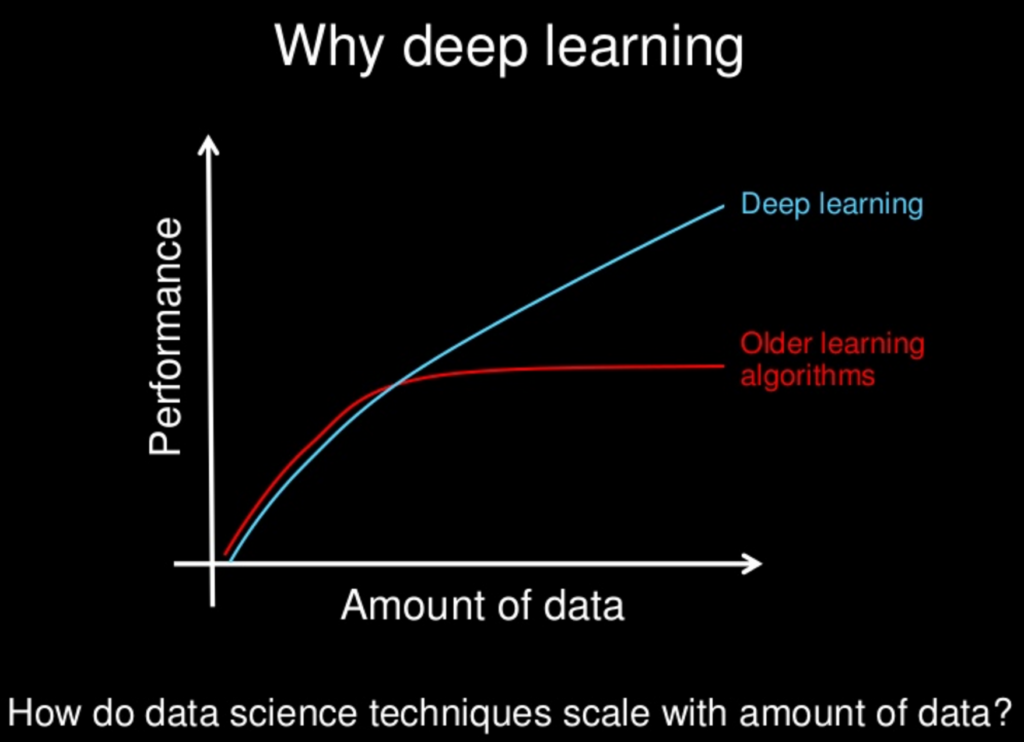Deep learning models tend to perform well with large amount of data whereas old machine learning models tend to stop improving after a saturation point and the learning curve flattens.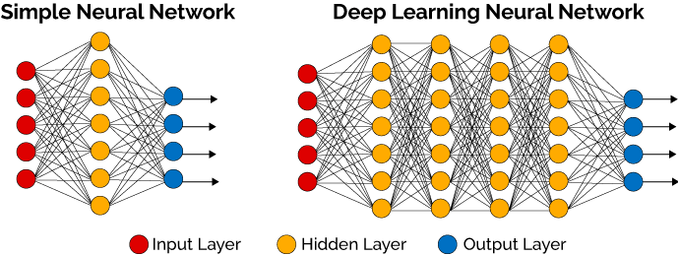## Neurons and Layers¶

Most neural network structures use some type of neuron. Many different kinds of neural networks exist, and programmers introduce experimental neural network structures all the time. An algorithm that is called a neural network will typically be composed of individual, interconnected units even though these units may or may not be called neurons. The name for a neural network processing unit varies among the literature sources. It could be called a node, neuron, cell, or unit.The artificial neuron receives input from one or more sources that may be other neurons or data fed into the network. This input is usually floating-point or binary. Often binary input is encoded to floating-point by representing true or false as $1$ or $0$. Sometimes the program also depicts the binary input as using a bipolar system with true as $1$ and false as $-1$.

### Weights¶

When input data comes into a neuron, it gets multiplied by a weight value that is assigned to this particular input. These weights start out as random values, and as the neural network learns more about the input data the network adjusts the weights based on any errors in categorization that the previous weights resulted in. This is training the neural network.

An artificial neuron multiplies each of these inputs by a weight. Then it adds these multiplications and passes this sum to an activation function. Some neural networks do not use an activation function. The following equation summarizes the calculated output of a neuron:

$$f(x, w) = \phi (\Sigma_{i} \theta_{i} \cdot x_{i}))$$

In the above equation, the variables $x$ and $\theta$ represent the input and weights of the neuron. The variable $i$ corresponds to the number of weights and inputs. You must always have the same number of weights as inputs. The neural network multiplies each weight by its respective input and feeds the products of these multiplications into an activation function that is denoted by the Greek letter $\phi$ (phi). This process results in a single output from the neuron.

### Types of Neurons¶

Not every neural network will use every kind of neuron. It is also possible for a single neuron to fill the role of several different neuron types.

There are usually four types of neurons in a neural network:

• Input Neurons - Each input neuron is mapped to one element in the feature vector.
• Hidden Neurons - Hidden neurons allow the neural network to be abstract and process the input into the output.
• Output Neurons - Each output neuron calculates one part of the output.
• Context Neurons - Holds state between calls to the neural network to predict.
• Bias Neurons - Work similar to the y-intercept of a linear equation.

### Context Neurons¶

Recurrent neural networks make use of context neurons to hold state. This type of neuron allows the neural network to maintain state. As a result, a given input may not always produce the same output. This inconsistency is similar to the workings of biological brains. Consider how context factors in your response when you hear a loud horn. If you hear the noise while you are crossing the street, you might startle, stop walking, and look in the direction of the horn. If you hear the horn while you are watching an action-adventure film in a movie theatre, you don't respond in the same way. Therefore, prior inputs give you the context for processing the audio input of a horn.

Time series is one application of context neurons. You might need to train a neural network to learn input signals to perform speech recognition or to predict trends in security prices. Context neurons are one way for neural networks to deal with time-series data. Figure 3.CTX shows how a neural network might arrange context neurons:

##### Figure 3.CTX: Neural Network with Context Neurons¶## Bias Neurons¶

Bias is just like an intercept added in a linear equation. It is an additional parameter in the Neural Network which is used to adjust the output along with the weighted sum of the inputs to the neuron. Moreover, bias value allows you to shift the activation function to either right or left, which may be critical for successful learning.

Consider this 1-input, 1-output network that has no bias:The output of the network is computed by multiplying the input (x) by the weight (w0) and passing the result through some kind of activation function (e.g. a sigmoid function.)

Here is the function that this network computes, for various values of w0:Changing the weight w0 essentially changes the "steepness" of the sigmoid. That's useful, but what if you wanted the network to output 0 when x is 2? Just changing the steepness of the sigmoid won't really work -- you want to be able to shift the entire curve to the right.

That's exactly what the bias allows you to do. If we add a bias to that network, like so:Now the output of the network becomes sig(w0*x + w1*1.0). Here is what the output of the network looks like for various values of w1:Having a weight of -5 for w1 shifts the curve to the right, which allows us to have a network that outputs 0when x is 2.

### What Is An Input Layer?¶

The input layer is responsible for receiving the inputs. These inputs can be loaded from an external source such as a web service or a csv file etc. There must always be one input layer in a neural network. The input layer takes in the inputs, performs the calculations via its neurons and then the output is transmitted onto the subsequent layers.

### What Is A Hidden Layer?¶

Hidden layers reside in-between input and output layers and this is the primary reason why they are referred to as hidden. The word “hidden” implies that they are not visible to the external systems and are “private” to the neural network. There could be zero or more hidden layers in a neural network. The larger the number of hidden layers in a neural network, the longer it will take for the neural network to produce the output and the more complex problems the neural network can solve.

### What Is An Output Layer?¶

The output layer is responsible for producing the final result. There must always be one output layer in a neural network. The output layer takes in the inputs which are passed in from the layers before it, performs the calculations via its neurons and then the output is computed.

## Activation Functions¶

Activation functions are functions that decide, given the inputs into the node, what should be the node’s output be? It is used to determine the output of neural network like yes or no. It maps the resulting values in between 0 to 1 or -1 to 1 etc. (depending upon the function).

Primary activation functions used

• Rectified Linear Unit (ReLU): Used for the output of hidden layers. Also can be used for regression output. Some gradients can be fragile during training and can die. It can cause a weight update that makes it never activate on any data point again. Simply saying that ReLu could result in Dead Neurons. In another words, For activations in the region $( x < 0)$ of ReLu, the gradient will be $0$ because the weights will not get adjusted during descent. That means, those neurons which go into this state will stop responding to variations in error/ input (simply because gradient is $0$, nothing changes). This is called "dying ReLu" problem.
• Exponential Linear Unit (ELU): very similiar to ReLU except it accepts negative inputs. Mostly a benefit in very deep netowrks with $40$ + layers. For $x > 0$, it can blow up the activation with the output range of $[0, \text_{inf}]$ "exploding gradient" problem.
• Leaky ReLU: Attempt to fix the “dying ReLU” problem by having a small negative slope (of $0.01$, or so)
• Parametric ReLU (PReLU): An extension of LeakyReLU but the slope in the nagative is learned via backpropagation.
• Maxout: Outputs the max of the inputs and is designed to be used in conjunction with the dropout regularization technique. The Maxout activation is a generalization of the ReLU and the leaky ReLU functions. It is a learnable activation function. Does not have its drawbacks (dying ReLU)
• Swish: Swish multiplies of the input $x$ with the sigmoid function for $x$. Swish is a smooth function. That means that it does not abruptly change direction like ReLU does near $x = 0$. Rather, it smoothly bends from $0$ towards values $< 0$ and then upwards again. This observation means that it’s also non-monotonic. Thus it does not remain stable or move in one direction, such as ReLU.
• Softmax: Used for the output of classification neural networks.
• Sigmoid: Used for the output of classification neural networks. Scales output from $0 - 1$. Towards either end of the sigmoid function, the $y$ values tend to respond very less to changes in $X$. It gives rise to a problem of “vanishing gradients”.
• TanH: Very similat to sigmoid usually preffered over sigmoid. Scales output from $-1 - 1$. The gradient is stronger for tanh than sigmoid (derivatives are steeper) but still has the “vanishing gradient” problem.
• Linear: Used for the output of regression neural networks (or 2-class classification).

Choosing an activation function

• For multi class classification us softmax
• RNN's do well with TanH
• For all other cases
• If you to improve try LeakyReLU
• Last resort try PReLU or Maxout
• Use Swish for very deep networks.

#### Rectified Linear Units (ReLU)¶

Introduced in 2000 by Teh & Hinton, the rectified linear unit (ReLU) has seen very rapid adoption over the past few years. Before the ReLU activation function, the programmers generally regarded the hyperbolic tangent as the activation function of choice. Most current research now recommends the ReLU due to superior training results. As a result, most neural networks should utilize the ReLU on hidden layers and either softmax or linear on the output layer. The following equation shows the straightforward ReLU function:

$$\phi(x) = \max(0, x)$$

We will now examine why ReLU typically performs better than other activation functions for hidden layers. Part of the increased performance is because the ReLU activation function is a linear, non-saturating function. Unlike the sigmoid/logistic or the hyperbolic tangent activation functions, the ReLU does not saturate to $-1$, $0$, or $1$. A saturating activation function moves towards and eventually attains a value. The hyperbolic tangent function, for example, saturates to $-1$ as $x$ decreases and $1$ as $x$ increases.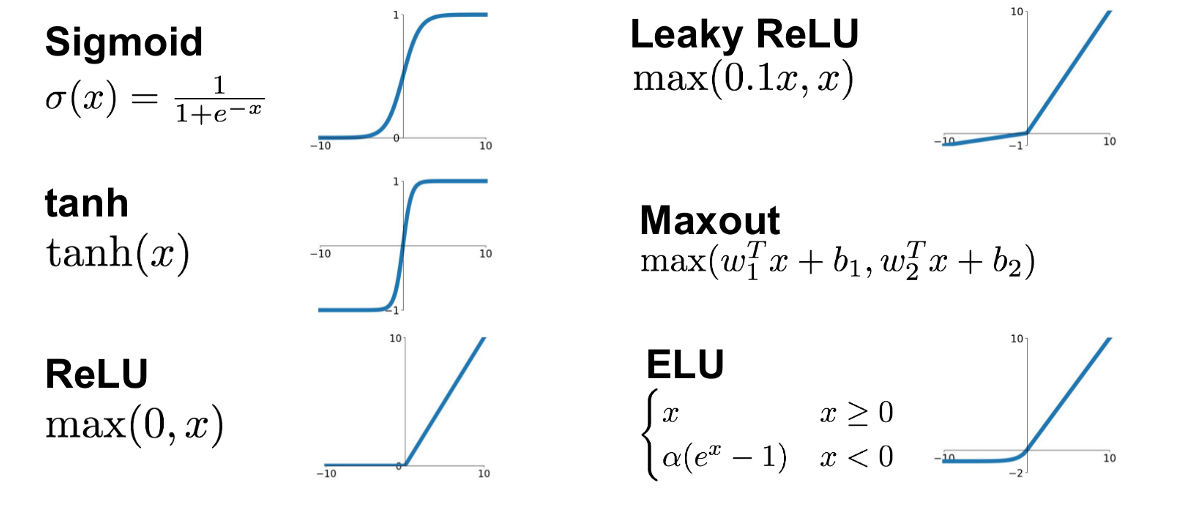Most current research states that the hidden layers of your neural network should use the ReLU activation.

## Forward Propagation¶

For Deep learning, several Neural Network layers are connected in feedforward or feedback style also called forward propagation to pass information to each other.

• Feedforward: This is the simplest type of ANN. The connections do not form a cycle and hence has no loops. The input is directly fed to output (in a single direction) through a series of weights. They are extensively used in pattern recognition. This type of organization is referred to as bottoms-up or top-down approach.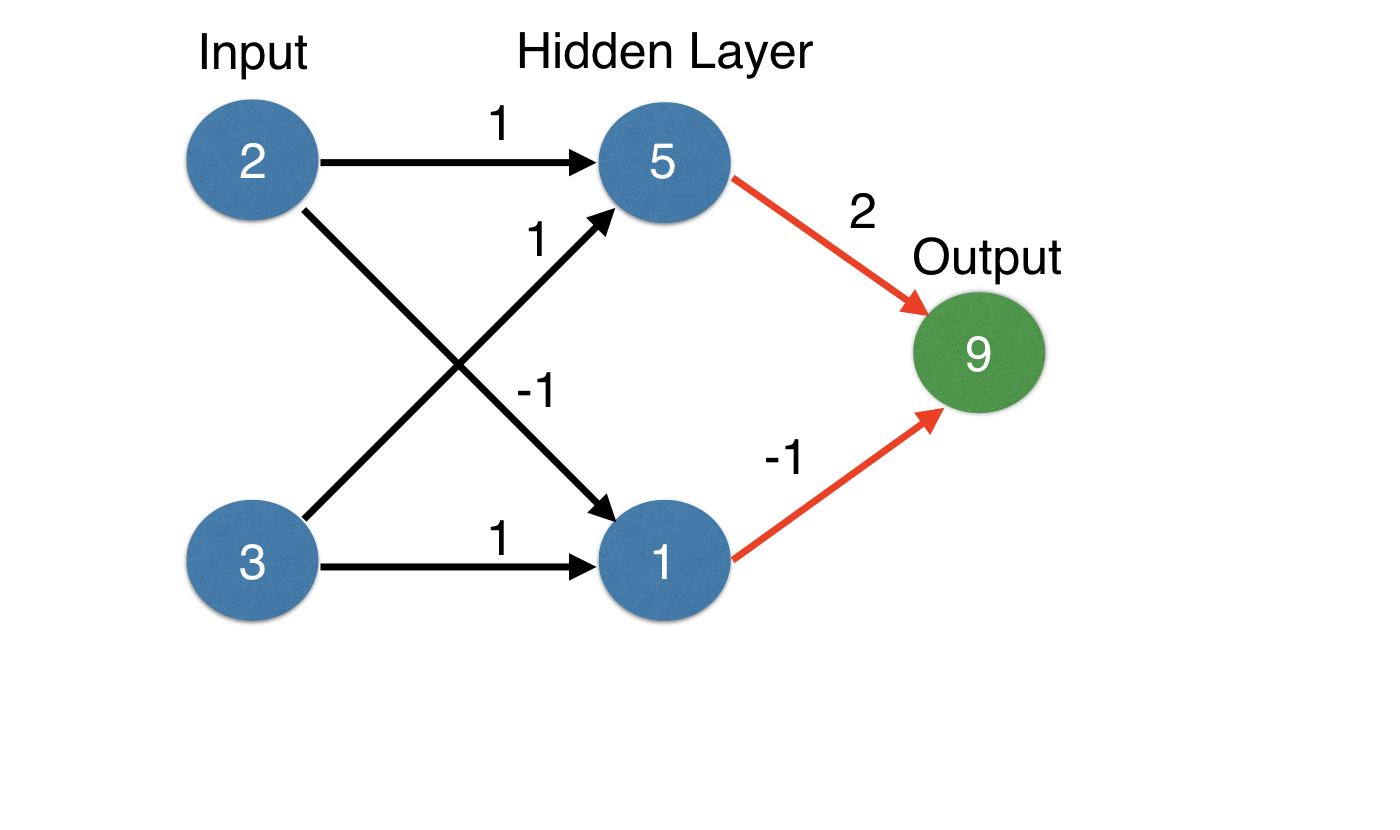• Dot product
• Forward propagation for one data point at a time
• Output is the prediction for that data point
$$y = \Sigma(input \ast weight) + bias$$

Feed forward example

In :
import numpy as np

input_data = np.array([2, 3])

print('input_data',input_data)

weights = {'node_0': np.array([1, 1]),
'node_1': np.array([-1, 1]),
'output': np.array([2, -1])}

node_0_value = (input_data * weights['node_0']).sum()
node_1_value = (input_data * weights['node_1']).sum()

input_data [2 3]

In :
hidden_layer_values = np.array([node_0_value, node_1_value])

print('hidden layer values',hidden_layer_values)

output = (hidden_layer_values * weights['output']).sum()

print('\noutput',output)

hidden layer values [5 1]

output 9


Sigmoid Activation Function Example

In :
def sigmoid(X):
return 1/(1+np.exp(-X))

input_data = np.array([-1, 2])

weights = {'node_0': np.array([3, 3]),
'node_1': np.array([1, 5]),
'output': np.array([2, -1])}

node_0_input = (input_data * weights['node_0']).sum()
node_0_output = sigmoid(node_0_input)

node_1_input = (input_data * weights['node_1']).sum()
node_1_output = sigmoid(node_1_input)

hidden_layer_output = np.array([node_0_output, node_1_output])
output = (hidden_layer_output * weights['output']).sum()

print('\nOutput',output)

Output 0.905271648220853


## Representation learning¶

• Deep networks internally build representations of patterns in the data
• Partially replace the need for feature engineering
• Subsequent layers build increasingly sophisticated representations of raw data
• Modeler doesn’t need to specify the interactions
• When you train the model, the neural network gets weights that find the relevant patterns to make better predictions

## The need for optimization¶

Predictions with multiple points

• Making accurate predictions gets harder with more points
• At any set of weights, there are many values of the error
• ... corresponding to the many points we make predictions for

## Loss function¶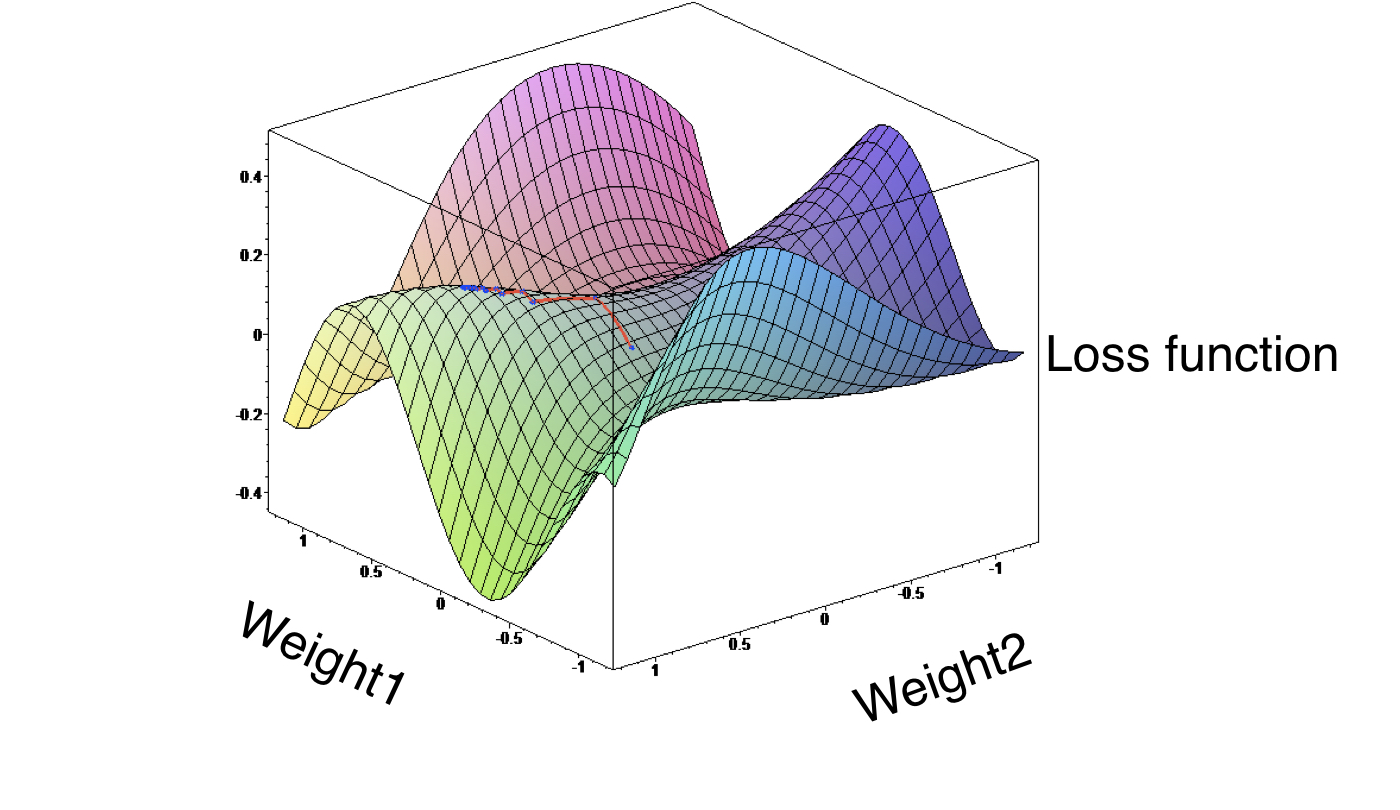A loss function or cost function is a function that maps an event or values of one or more variables onto a real number intuitively representing some cost associated with the event. An optimization problem seeks to minimize a loss function. An objective function is either a loss function or its negative in which case it is to be maximized. The Loss function computes the error for a single training example while the Cost function is the average of the loss functions for all the training examples.

• Aggregates errors in predictions from many data points into single number
• Measure of model’s predictive performance

Lower loss function value means a better model

• Goal: Find the weights that give the lowest value for the loss function• $X$ = parameter to optimize
• $y$ = loss or cost function
• The goal is to minimize the function. We need to find that value of $X$ that produces the lowest value of $y$.

Gradient descent refers to the calculation of a gradient on each weight in the neural network for each training batch. The gradient of each weight will give you an indication about how to modify each weight to achieve the expected output. If the neural network did output exactly what was expected, the gradient for each weight would be $0$, indicating that no change to the weight is necessary.

The gradient is the derivative of the error function at the weight's current value. The error function measures the distance of the neural network's output from the expected output.

With respect to the error function, the gradient is essentially the partial derivative of each weight in the neural network. Each weight has a gradient that is the slope of the error function. A weight is a connection between two neurons. Calculating the gradient of the error function allows the training method to determine whether it should increase or decrease the weight. In turn, this determination will decrease the error of the neural network. Many different training methods called optimizers utilize gradients. In all of them, the sign of the gradient tells the neural network the following information:

• Zero gradient: The weight is not contributing to the error of the neural network.
• Negative gradient: The weight should be increased to achieve a lower error.
• Positive gradient: The weight should be decreased to achieve a lower error.

The learning rate is an important concept for Gradient descent. This is the size of the steps taken to reach the functions minium. Setting the learning rate can be complex:

Too low of a learning rate will usually converge to a good solution; however, the process will be very slow. Too high of a learning rate will either fail outright, or converge to a higher error than a better learning rate.

Common values for learning rate are: $0.1$, $0.01$, $0.001$, etc.

### Slope calculation example¶

To calculate the slope for a weight, need to multiply:

• Slope of the loss function with respect to the value at the node we feed into
• The value of the node that feeds into our weight
• Slope of the activation function with respect to the value we feed into

#### Here we calculate slopes and update weights¶

In :
weights = np.array([1, 2])
input_data = np.array([3, 4])

target = 6
learning_rate = 0.01

preds = (weights * input_data).sum()
error = preds - target
print(error)

5

In :
gradient = 2 * input_data * error

[30 40]

In :
weights_updated = weights - learning_rate * gradient
preds_updated = (weights_updated * input_data).sum()
error_updated = preds_updated - target
print(error_updated)

2.5


## Backpropagation¶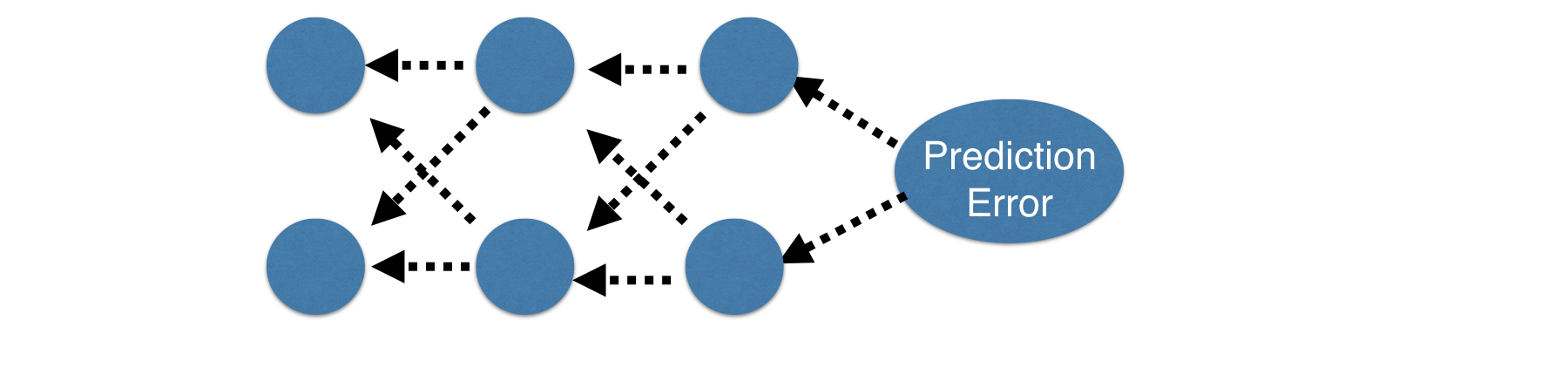Back-propagation is the essence of neural net training. It is the practice of fine-tuning the process of updating the weights of a neural net based on the error rate (i.e. loss) obtained in the previous epoch (i.e. iteration). Proper tuning of the weights ensures lower error rates, making the model reliable by increasing its generalization.

• Allows gradient descent to update all weights in neural network (by getting gradients for all weights)
• Comes from chain rule of calculus

Backpropagation process

• Start at some random set of weights
• Use forward propagation to make a prediction
• Use backward propagation to calculate the slope of the loss function with respect to each weight
• Multiply that slope by the learning rate, and subtract from the current weights
• Keep repeating the process until we get to a flat part.